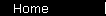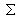### Covariance

A statistical measure of correlation of the fluctuations of two different quantities. In finance, covariance is applied to the annual rates of return of different investments, to measure the correlation of their year-to-year fluctuations in performance.

The definition is

Cov(r1, r2) = 1/n *(r1 i - r1 ave) * (r2 i - r2 ave)

where the terms r1 i and r2 i are actual values of the annual rates of return of two investments, taken over several years, n is the total number of values of r1 i and r2 i used, and r1 ave and r2 ave are the average values of r1 i and r2 i.

See the article on the efficient frontier for a description of how covariance is related to asset allocation.

 home  |  glossary  |  calculator  |  about us  |  books